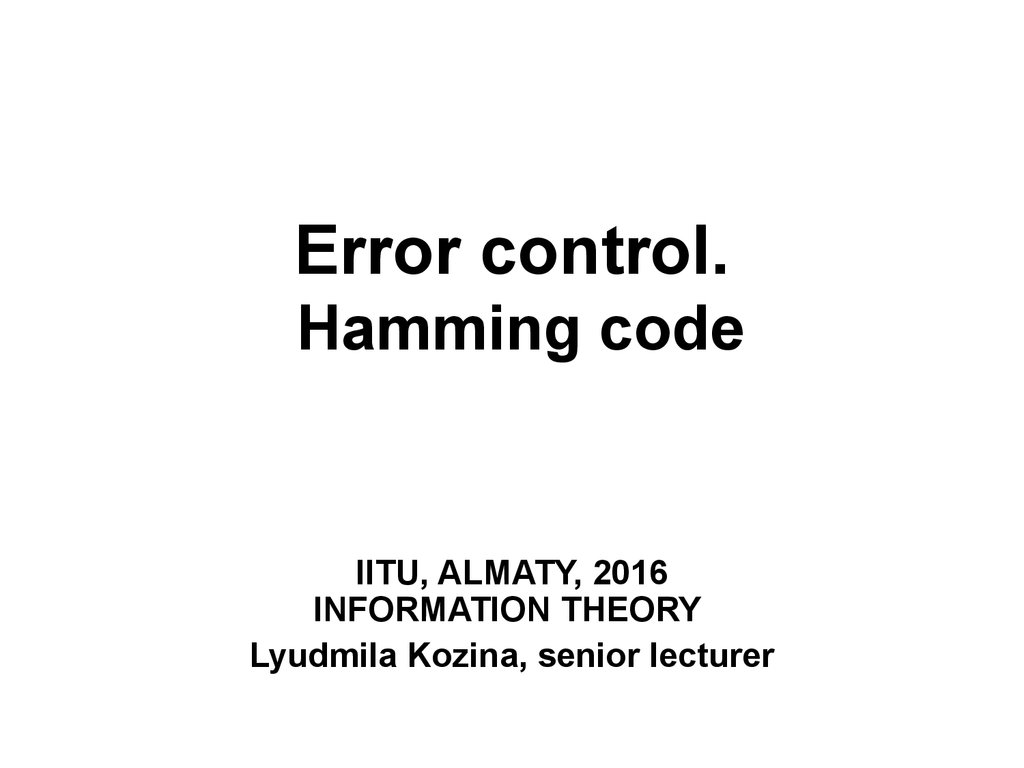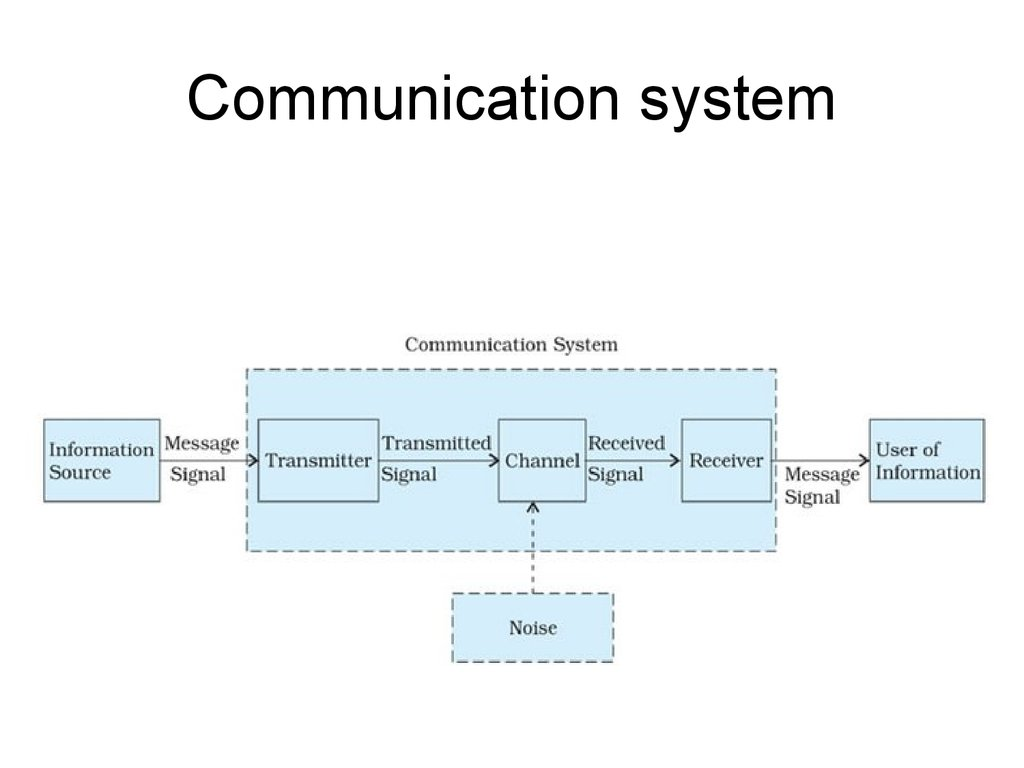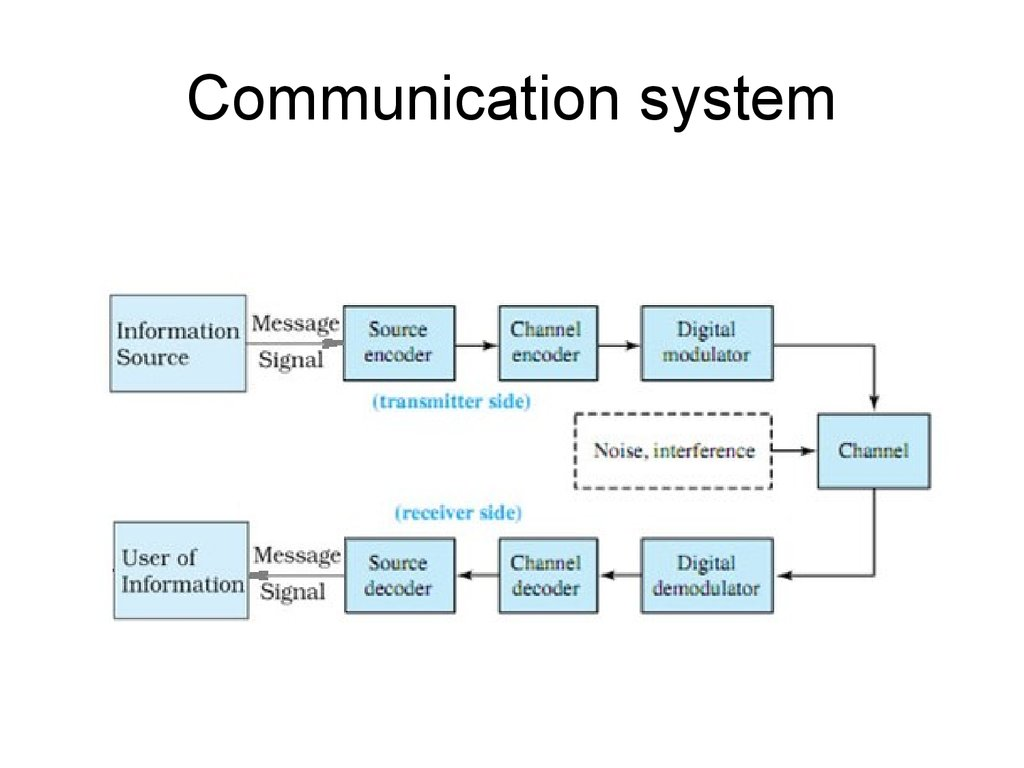# Error control. Hamming code

## 1. Error control. Hamming code

IITU, ALMATY, 2016
INFORMATION THEORY
Lyudmila Kozina, senior lecturer

## 4. Error control

• In information theory and coding theory with applications
in computer science and telecommunication error
control are techniques that enable reliable delivery of
digital data over unreliable communication channels.
Types of error control:
• Error detection is the detection of errors caused by
noise or other impairments during transmission from the
• Error correction is the detection of errors and
reconstruction of the original, error-free data.

## 5. Main idea

• The general idea for achieving error detection and
correction is to add some redundancy (i.e., some
extra data) to a message, which receivers can use to
check consistency of the delivered message, and to
recover data determined to be corrupted.

## 7. Error control

Different techniques of coding:
• Block code
• Convolutional code

## 8. Block codes

k - length of each block before coding
n - length of each block after coding
such codes are denoted (n, k)
as before n > k
code rate:
R=k/n

## 9. Block codes

Blocks:
• Data bits – information
• Parity bits – redundant

## 10. Example

Parity bit:
• 0 – if number of “1” in code combination is
even
• 1 – if number of “1” in code combination is
odd
Example
• 101 – code combination before coding
• 1010 - code combination after coding

## 11. Different combinations

Types of code combinations after a
channel:
• permissible (allowable) combinations –
code combination without error(s)
• prohibited (not allowable)
combinations - code combination with
error(s)

## 12. Detection or correction?

• Hamming distance between two strings of
equal length is the number of positions at which
the corresponding symbols are different.
• In another way, Hamming distance measures
the minimum number of substitutions required to
change one string into the other, or the minimum
number of errors that could have transformed
one string into the other.

## 13. Hamming distance: examples

"karolin" and "kathrin" is 3.
"karolin" and "kerstin" is 3.
1011101 and 1001001 is 2.
2173896 and 2233796 is 3.
• For binary strings a and b the Hamming distance
is equal to the number of ones in a XOR b.

## 14. Example 1: Hamming distance=1

• When d=1 all code combinations are
allowable and any mistake will cause the
transition to another allowable code
combination. Which means that no error
can be detected. For example, when n=3,
allowable combinations form the following
set:
000 001 010 011 100 101 110 111

## 15. Example 2: Hamming distance=2

• With Hamming code distance d=2 there is
no one from allowable code words which
could transform to another. These are
combinations, so errors can be detected,
but not corrected. For example, suppose
n=3 as before.
allowable
000
011
101
110
not allowable
001
010
100
111

## 16. Example 3: Hamming distance=3

• In this example Hamming distance is
enough for not only error detection, but
also error correction. Every allowable
combination has set of not allowable.
allowable
not allowable
000
001
010
100
111
011
101
110

## 17. Basic formulas

• Detect errors of multiplicity r:
dmin >= r+1
• Correct errors of multiplicity s:
dmin >= 2s+1
• To detect errors of multiplicity r and to correct
errors of multiplicity s (general formula):
dmin >= s+r+1

## 18. Hamming code

• Hamming codes are a family of linear
error-correcting codes that generalize the
Hamming (7,4) - code invented by Richard
Hamming in 1950.
• Error detection &
error correction

## 19. Main ideas

• Hamming was interested in two problems at
once:
– increasing the distance as much as possible
– at the same time increasing the code rate as much as
possible.
• The key to all of his systems was to have the
parity bits overlap, such that they managed to
check each other as well as the data.

## 20. Structure rule of Hamming codes

For each integer r ≥ 2 there is a code with block
length n = 2^r−1 and message length k = 2^r−r−1.
(n, k)=(2^r−1, 2^r−r−1)
For example, (7, 4), (15, 11), (31, 26), etc
Parity bits Total bits Data bits
(r)
(n)
(k)
Name
Rate
3
7
4
Hamming(7,4)
4/7 ≈ 0.571
4
15
11
Hamming(15,11)
11/15 ≈ 0.733
5
31
26
Hamming(31,26)
26/31 ≈ 0.839

## 21. Hamming (7,4) code

• In 1950, Hamming introduced the (7,4)
Hamming code. It encodes 4 data bits into
7 bits by adding three parity bits.
• (i1, i2, i3, i4) -> (i1, i2, i3, i4, r1, r2, r3),
i – data bits and r – parity bits
• It can detect and correct single-bit errors –
SEC (single-error correcting ).

## 22. Encoding Hamming(7,4)

• The key thing about Hamming Codes is
that any given bit is included in a unique
set of parity bits.
• r1 = i1 XOR i2 XOR i3
• r2 = i2 XOR i3 XOR i4
• r3 = i1 XOR i2 XOR i4

## 23. Decoding (7,4)

• Decoder gets a codeword (i1, i2, i3, i4, r1, r2, r3),
where every bit can be an error bit (including data and
parity bits).
• The pattern of errors, called the error syndrome,
identifies the bit in error.
• S1 = r1 XOR i1 XOR i2 XOR i3
• S2 = r2 XOR i2 XOR i3 XOR i4
• S3 = r3 XOR i1 XOR i2 XOR i4
• S = (s1, s2, s3) - error syndrome

Error syndrome
Error bit
000
No error
001
r3
010
r2
011
i4
100
r1
101
i1
110
i3
111
i2

## 25. Example 1: an error in data bit

Combination before encoding: 1001
Combination after encoding: 1001110
Single random error: i4
Combination with error: 1000110
Decoding syndrome: 011 -> i4
Decoding (error correction): 1001110
After decoding 1001
25

## 26. Example 2: an error in parity bit

Combination before encoding: 1001
Combination after encoding: 1001110
Single random error: r2
Combination with error: 1001100
Decoding syndrome: 010 -> r2
Decoding (error correction): 1001110
After decoding 1001
26

## 27. General algorithm

• To compare different approaches consider
Hamming(7,4) as example.
• However this general algorithm can be
used for any length codewords.
• In the example:
Input: 4-bit code word x1…x4
27

## 28. Input codeword

• Row 1 – number of position in the codeword
• Row 2 – bit notation
• Row 3 – bit value
(so example codeword is “1001”)

## 29. Number of parity bits

• In general, the number of parity bits in the
codeword is equal to the binary logarithm
of the number of bits of the codeword
(including parity bits) in rounded up to the
nearest integer:
r ≈ log2(n)
• In the example, r = log2(7) ≈ 3

## 30. Addition of parity bits

• Number of positions are integer powers of 2: 0, 1,
2, etc: 2^0, 2^1, 2^2, etc.
• Now we have 7-bit word with 4 data bits and 3
parity bits.
• Initially parity bits are set to zero.

## 31. Transformation matrix

• Add to table 3 rows (number of parity bits) with a
transformation matrix.
• Each row is one parity bit (top down), each column is
one bit of codeword.

## 32. Transformation matrix

• Each column of a transformation matrix is a binary
number of this column, but bit order is reverse: the
least significant bit is on the top line, a senior - at the
bottom.
• For example, 3rd column is “110” corresponding to the
binary representation of the number “3”: 011.

## 33. Calculation of parity bits

r0 = (1·0+0·0+1·1+0·0+1·0+0·0+1·1) mod 2 = 2 mod 2 = 0
r1 = (0·0+1·0+1·1+0·0+0·0+1·0+1·1) mod 2 = 2 mod 2 = 0
r2 = 0·0+0·0+0·1+1·0+1·0+1·0+1·1 =1
• The resulting control bits inserted into codeword instead of
standing there before zeros.
• Hamming coding is completed. The resulting code word 0011001

## 34. Decoding

• Algorithm of decoding is absolutely
identical to encoding algorithm.
• Goal of decoding is get a syndrome
matrix.
• As before the syndrome matrix (0,0,0)
indicates a codeword without error, any
other – with error.
• For example, change one of the bits (6th bit) to show an error and its correction.

## 35. Decoding

s0 = (1*0+0*0+1*1+0*1+1*0+0*1+1*1) mod 2 = 2 mod 2 = 0
s1 = (0*0+1*0+1*1+0*1+0*0+1*1+1*1) mod 2 = 3 mod 2 = 1
s2 = (0*0+0*0+0*1+1*1+1*0+1*1+1*1) mod 2 = 3 mod 2 = 1
Syndrome matrix is (0,1,1)

## 36. Decoding

• Syndrome matrix is a binary number of error
position.
• In example s = 011 and decimal
representation of “110” is “6”, so error
position = 6.

37

## 38. References

• Arndt C.
Information Measures: Information and its
Description in Science and Engineering.
• Thomas Cover. Elements Of Information
Theory.